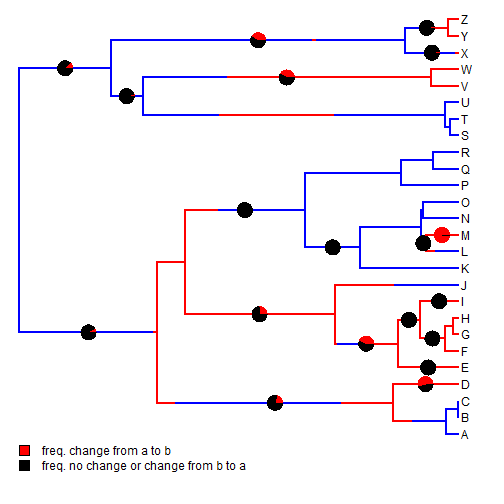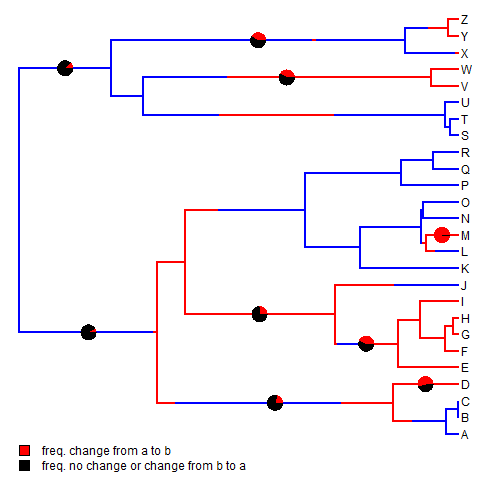## Saturday, November 7, 2015

### Computing the frequency of changes along edges of a set of stochastic map trees

In response to a recent R-sig-phylo thread I thought it might be useful to post code demonstrating how to compute the relative frequency of changes of particular types along edges in a posterior sample from the method of stochastic mapping.

``````## load libraries
library(phytools)
## here is our data
tree
``````
``````##
## Phylogenetic tree with 26 tips and 25 internal nodes.
##
## Tip labels:
##  A, B, C, D, E, F, ...
##
## Rooted; includes branch lengths.
``````
``````x
``````
``````##   A   B   C   D   E   F   G   H   I   J   K   L   M   N   O   P   Q   R
## "a" "a" "a" "b" "b" "b" "b" "b" "b" "a" "a" "a" "b" "a" "a" "a" "a" "a"
##   S   T   U   V   W   X   Y   Z
## "a" "a" "a" "b" "b" "b" "b" "b"
``````
``````## obtain stochastic maps:
trees<-make.simmap(tree,x,model="ER",nsim=100)
``````
``````## make.simmap is sampling character histories conditioned on the transition matrix
##
## Q =
##           a         b
## a -2.818303  1.409151
## b  1.409151 -2.818303
## (estimated using likelihood);
## and (mean) root node prior probabilities
## pi =
##   a   b
## 0.5 0.5
``````
``````## Done.
``````

Now let's do our analysis. The following function computes for a given edge (by row in `tree\$edge`, but we could easily modify to be by node number) the relative frequency of trees in which the state at the start of the edge is `"a"` and the state at the end is `"b"`.

``````## this function computes for a given edge
foo<-function(edge,trees,states){
obj<-sapply(trees,function(x,e,s)
if(names(x\$maps[[e]])==s&&names(x\$maps[[e]])[length(x\$maps[[e]])]==s) TRUE
else FALSE,e=edge,s=states)
sum(obj)/length(obj)
}
``````

We can use it by iterating over edges:

``````edge.probs<-sapply(1:nrow(tree\$edge),foo,trees=trees,states=c("a","b"))
edge.probs
``````
``````##   0.09 0.21 0.00 0.00 0.00 0.00 0.00 0.53 0.00 0.27 0.43 0.02 0.01 0.01
##  0.00 0.00 0.00 0.00 0.01 0.00 0.01 0.01 0.00 0.00 0.02 0.00 0.97 0.00
##  0.00 0.00 0.00 0.00 0.00 0.00 0.00 0.13 0.05 0.00 0.00 0.00 0.00 0.00
##  0.41 0.00 0.00 0.40 0.03 0.03 0.00 0.00
``````

Finally, let's visualize the results on a 'representative' stochastic map:

``````plot(trees[],colors=setNames(c("blue","red"),c("a","b")),
ylim=c(-2,Ntip(tree)))
par(fg="transparent") ## just for clarity
threshold<-0 ## threshold frequency above which we want to plot it
edgelabels(edge=which(edge.probs>threshold),
pie=edge.probs[which(edge.probs>threshold)],
piecol=c("red","black"),cex=0.8)
par(fg="black")
add.simmap.legend(colors=setNames(c("red","black"),c("freq. change from a to b",
"freq. no change or change from b to a")),prompt=FALSE,x=0,y=0)
``````Or we can change the threshold to `0.05`:

``````plot(trees[],colors=setNames(c("blue","red"),c("a","b")),
ylim=c(-2,Ntip(tree)))
par(fg="transparent") ## just for clarity
threshold<-0.05 ## threshold frequency above which we want to plot it
edgelabels(edge=which(edge.probs>threshold),
pie=edge.probs[which(edge.probs>threshold)],
piecol=c("red","black"),cex=0.8)
par(fg="black")
add.simmap.legend(colors=setNames(c("red","black"),c("freq. change from a to b",
"freq. no change or change from b to a")),prompt=FALSE,x=0,y=0)
``````BTW, for this example the data were simulated as follows:

``````tree<-pbtree(n=26,tip.label=LETTERS,scale=1)
Q<-matrix(c(-1,1,1,-1),2,2)/2
rownames(Q)<-colnames(Q)<-letters[1:2]
x<-sim.history(tree,Q,anc="a")\$states
``````